IdentifiantMot de passe
Mot de passe oublié ?

Inscrivez-vous gratuitement
pour pouvoir participer, suivre les réponses en temps réel, voter pour les messages, poser vos propres questions et recevoir la newsletter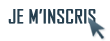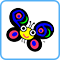Discussion :

Récupérer une date à partir d'un format UNIX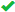Sujet :

1.Récupérer une date à partir d'un format UNIX
Salut,

Donc j'ai des fichiers dont leur nom contient une date. Ce nom de fichier a été créé à l'aide du format UNIX disponible dans un fichier de configuration.

Par exemple :
config-%Y%m%d => config-20090204
Ce que je voudrais, c'est pouvoir récupérer la date (en secondes ou autre).

Le problème est que le format du nom de fichier peut varier. Il me faudrait donc une fonction qui accepte : la chaîne, le format à partir duquel elle a été générée et me retourne la date résultante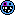.

Si j'ai bien regardé ni TimeDate ni Date::Manip ne le propose...Répondre avec citation   0  0

2.Comprends pas... Tu veux récupérer la date qui est dans le nom de fichier ? Ou bien récupérer la date du jour ou de l'instant t ?Répondre avec citation   0  0

3.Je souhaite récupérer la date qui est dans le nom de fichier mais comme son format peut changer je cherche une fonction qui me donnerais la date contenu dans le nom du fichier en se basant sur la chaîne de format l'ayant créé...

Voici quelques exemples :

config_20080204.cfg => config_%Y%m%d.cfg
config_2008-02-04.cfg => config_%Y-%m-%d.cfg
config-4-2-09.cfg => config-%e-%L-%y.cfg
Bref ça peut-être tout et n'importe quoi...Répondre avec citation   0  0

4.Code Perl : Sélectionner tout - Visualiser dans une fenêtre à part
 123 my \$file = 'config-20090204.txt'; \$file =~ m/(\d{8})/; print \$1;Répondre avec citation   0  0

5.Peut-être le module http://search.cpan.org/~sbeck/Date-M...Date/Manip.pod, avec la fonction UnixDate ?
 Code : Sélectionner tout - Visualiser dans une fenêtre à part
 123 @date = UnixDate(\$date,@format); \$date = UnixDate(\$date,@format);Répondre avec citation   0  0

6.J'ai aussi bien essayé TimeDate (Date::Parse) que Date::Manip mais dès qu'il y a une chaîne qu'ils ne peuvent interpréter comme une date leurs fonctions ne retourne aucun résultat.Répondre avec citation   0  0

7.Bon n'ayant rien trouvé sur le net j'ai créé mon petit script. Ce n'est pas totalement fini mais ça à l'air de fonctionner pour l'instant.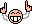Code : Sélectionner tout - Visualiser dans une fenêtre à part
 123456789101112131415161718192021222324252627282930313233343536373839404142434445464748495051525354555657585960616263646566676869707172737475767778798081828384858687888990919293949596979899100101102103104105106107108109110111112113114115116117118119 #!/usr/bin/env perl   use strict; use warnings; use diagnostics;       my %unix_time_regex = ( "a" => qr/(\w{3})/, "A" => qr/(\w+)/, "b" => qr/(\w{3})/, "B" => qr/(\w+)/, "c" => qr/((:?\d{2}\/\d{2}\/\d{2})\s+(:?\d{2}:\d{2}:\d{2}))/, "C" => qr/(\w{3}\s+\w{3}\s+\d{1,2}\s+(:?\d{2}:\d{2}:\d{2})\s+(:?.*)\s+\d{4})/, "d" => qr/(\d{2})/, "e" => qr/(\d{1,2})/, "D" => qr/(\d{2}\/\d{2}\/\d{2})/, "G" => qr/(\d+)/, "h" => qr/(\w{3})/, "H" => qr/(\d{2})/, "I" => qr/(\d{2})/, "j" => qr/(\d{3})/, "k" => qr/(\d{1,2})/, "l" => qr/(\d{1,2})/, "L" => qr/(\d{1,2})/, "m" => qr/(\d{2})/, "M" => qr/(\d{2})/, "n" => qr/(\n)/, "o" => qr/(\d{1,2}(:?st|nd|rd|th))/, "p" => qr/(AM|PM)/, "P" => qr/(am|pm)/, "q" => qr/([1-4])/, "r" => qr/((:?\d{2}:\d{2}:\d{2})\s+(:?AM|PM))/, "R" => qr/(\d{2}:\d{2})/, "s" => qr/(\d+)/, "S" => qr/(\d{2})/, "t" => qr/(\t)/, "T" => qr/(\d{2}:\d{2}:\d{2})/, "U" => qr/(\d+)/, "w" => qr/([0-6])/, "W" => qr/(\d+)/, "x" => qr/(\d{2}\/\d{2}\/\d{2})/, "X" => qr/(\d{2}:\d{2}:\d{2})/, "y" => qr/(\d{2})/, "Y" => qr/(\d{4})/, "Z" => qr/(\w+)/, "z" => qr/([+-]?\d{4})/);     while (my \$line = ) { chomp \$line;   my (\$string, \$format) = split(', ', \$line);   my @fmt_array = split(/\%/, \$format); my @reg_array = ();   foreach my \$str_slice (@fmt_array) { print "\$str_slice\n"; if (defined(\$unix_time_regex{\$str_slice})) { \$str_slice = \$unix_time_regex{\$str_slice}; } push(@reg_array, \$str_slice); }   my \$regex = join('',@reg_array); print "\$regex\n";   if (\$string =~ m/\$regex/) { print "Match \'\$string\' with \'\$format\': \$1\n"; } }       __DATA__ Wed, %a Wednesday, %A Feb, %b February, %B 02/04/09 23:13:52, %c Wed Feb 4 23:13:52 CET 2009, %C 04, %d 4, %e 02/04/09, %D 1517, %G Feb, %h 23, %H 11, %I 035, %j 23, %k 11, %l 2, %L 02, %m 13, %M 4th, %o PM, %p pm, %P 1, %q 11:13:52 PM, %r 23:13, %R 1233785632, %s 52, %S , %t 23:13:52, %T 5, %U 3, %w 5, %W 02/04/09, %x 23:13:52, %X 09, %y 2009, %Y CET, %Z +0100, %z
À chaque symbole est associé une expression régulière, celle-ci puis le tout est recréé à partir du format et il ne reste plus qu'à "matcher". Je ne sais pour l'instant pas matcher le symbole '%' mais dans mon cas ça n'est pas trop grave.

Si vous avez quelques conseilles pour améliorer les expressions régulières ou autre n'hésitez pas.

Il me faut maintenant à partir de tout ça créer une date cohérente et la retourner.Répondre avec citation   0  0

8.As-tu essayé le module DateTime::Format::Strptime ?
Il fait exactement ce que tu veux.

--
JedaïRépondre avec citation   0  0

9.Wow merci, j'avais beau parcourir CPAN et le net dans tout les sens et je ne suis jamais tombé sur ce module... Je cherchais sûrement avec de mauvais mots clé...

En tout cas merci, ça va me faciliter la vieRépondre avec citation   0  0

+ Répondre à la discussion
Cette discussion est résolue.
 Actualités FAQs PERL TUTORIELS PERL LIVRES PERL TELECHARGEZ QUIZZ